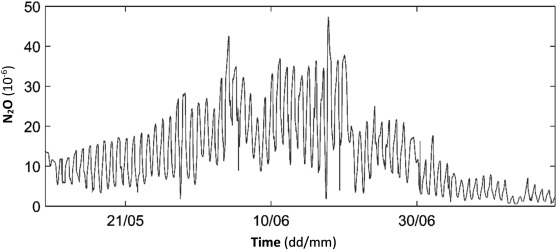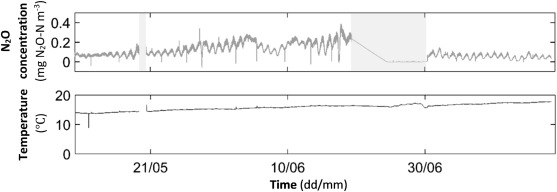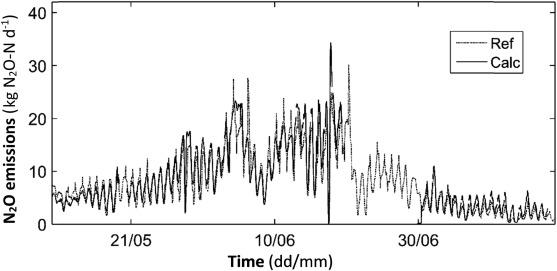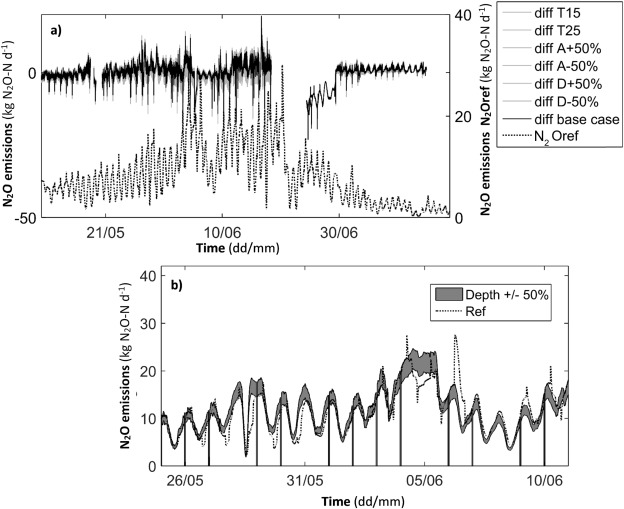## Abstract

Total nitrous oxide (N2 O) emissions from a conventional active sludge process were calculated using a mathematical emission model and dissolved N2 O in the water phase by a N2 O water sensor. Calculated emissions showed good agreement with measured emissions based on concentrations and airflow in the off-gas ventilation system from the covered process. Various tests for a wide range of potentially affecting parameters indicated robust and reliable emission estimations by the model. Thus, common uncertainties in input data would still provide good emission estimations. Measurements of dissolved N2 O required low maintenance and N2 O emission monitoring based on such measurements might therefore be considered as a significant practical improvement. This would be advantageous especially in non-enclosed wastewater treatment plants (WWTPs) otherwise requiring complex off-gas measurements. As the sensor is connected directly to the WWTPs monitoring and control system, emissions calculations could be incorporated.

## Keywords

GHG emissions ; Wastewater treatment ; Nitrous oxide

## 1. Introduction

Greenhouse gas (GHG) emissions are of increasing concern within all sectors of society and thus also for wastewater treatment. Even though, treating wastewater is essentially positive for the environment as both organic matter, nutrients and other harmful substances that might cause damage to the receiving waters are removed, GHG emissions from the treatment processes have a negative impact on the environment. Special attention is given to nitrous oxide (N2 O), which is a highly potent GHG (298 times more potent than CO2 ) (IPCC, 2007 ). This has gained more and more attention in recent years e.g. in Sweden (Arnell, 2013 , Czepiel et al., 1995 , Jönsson et al., 2015 , Tumlin et al., 2014 , Westling, 2011 , Wunderlin et al., 2012  and Yoshida et al., 2014 ).

The formation of N2 O is a common problem for all biological nitrogen removal processes for which general mechanisms of N2 O emissions have been understood but actual emissions are site-specific and depend on several overlapping factors. The understanding of such emissions is important in order to reduce these emissions at maintained nitrogen removal efficiency. In wastewater treatment plants (WWTPs) N2 O can be produced at different stages of the complex nitrogen cycle. Partly in connection with the first steps in nitrification, oxidation of ammonium/ammonia to nitrite (${\textstyle NO_{2}^{-}}$ ), and partly during denitrification of nitrite to nitrogen gas (N2 ). It is therefore not surprising that N2 O is emitted from various stages of the wastewater treatment.

Despite much research in recent years, there are still large uncertainties in terms of GHG emissions from the treatment of municipal wastewater handling (Jönsson et al., 2015 ). Published measurements on GHG emissions from different wastewater treatment plants across the world show great spread (Chandran, 2010 , Foley et al., 2010 , Foley et al., 2011 , Kampschreur et al., 2009  and Staaf and Bergström, 2012 ). This due to varying processes measured but also due to problems in measuring emissions adequately.

Especially short-term concentration measurements at single locations above various treatment processes imply significant uncertainties in emission estimations. Therefore, more developed measuring methods such as using floating chambers to collect all emissions from a defined surface area have been developed and used e.g. by the authors and others (Arnell, 2013 , Jönsson et al., 2015 , Stenström et al., 2013 , Stenström et al., 2014 , Tjus et al., 2011  and Yang et al., 2015 ). In addition, some underground WWTPs in Scandinavia have been used to verify such measuring techniques and to gain more understanding of emission causing factors by full-scale tests (e.g. Carlsson, 2012  and Jönsson et al., 2015 ).

Even though, measuring instruments and equipment to quantify these emissions have been improved, intensive handling of the equipment or expensive automatic analyses generally imply that only time-limited measurements are possible especially in open WWTPs. These measurements, however, provide only limited information about emissions dynamics. Simpler and robust measuring techniques could thus significantly improve the measuring of N2 O emissions and increase the understanding and mitigation work to reduce emissions from WWTPs. This would also reduce the uncertainty and, often, questionable estimation of emissions caused by the use of fixed ratio values from literature.

One approach is to measure dissolved N2 O in the water phase, as process changes and their impact on the N2 O formation could then be directly monitored. However, as N2 O is both produced and consumed, the emission estimation requires an appropriate modelling of the actual emissions to the process off-gas. The authors have been using the first generation of commercially available sensors alongside other measuring techniques in a number of projects (e.g. Jönsson et al., 2015 , Stenström et al., 2013 , Stenström et al., 2014 , Tjus et al., 2011  and Yang et al., 2015 ). Although the sensor could measure dissolved N2 O, the sensor was too fragile and unstable to be used on a regular basis in full-scale processes.

This paper is to show that previous efforts and experiences from these projects and further improvements of dissolved N2 O-sensors make it possible to estimate total emissions of N2 O to air for both aerated and non-aerated zones by combining the measurements with an established emission model. During a measurement campaign at Käppala WWTP, Sweden, continuous dissolved N2 O measurements combined with an emission model was compared to simultaneous off-gas measurements in a compete enclosed process environment of a full-scale activated sludge process.

## 2. Materials and methods

### 2.1. Käppala WWTP

Swedens third largest WWTP Käppala is situated underground on the island of Lidingö. It serves 11 municipalities in the Stockholm region and operates as an Activated Sludge Process in the UCT-configuration with mechanical, biological and chemical treatment and an ammonium feedback control, which implies varying dissolved oxygen concentrations in the process. Discharge permits during the measuring campaign were ≤10 mg L−1 for Nitrogen, ≤0.3 mg L−1 for Phosphorous and ≤8 mg L−1 for BOD7 .

The measurement campaign was carried out during three months in one of the newer treatment lines (Block BB11) at Käppala WWTP. BB11 comprises seven zones of which the three zones in the middle are aerated. Earlier measurements of N2 O emissions at Käppala WWTP in the same treatment line indicated emissions of 5.5 tones N2 O per year from biological treatment processes representing 0.3% of the incoming nitrogen.

### 2.2. Off-gas N2 O measurements and emission calculations

Reference measurements of N2 O in the off-gas were conducted in the ventilation shaft. As the biological treatment is located underground in an enclosed space, all emissions from the treatment line pass through the ventilation shaft. Fresh air is supplied from the subsequent sedimentation (ES11), passing BB11 and is then emitted by the 149.5 m high chimney. Weekdays between 4:30 p.m. and 06:00 a.m. and on weekends, the ventilation is operated constant at about 60% of the normal constant airflow during working hours. The airflow in the ventilation were measured several times to 29,200 m3  h−1 during weekdays, daytime. At reduced ventilation, the flow is about 17,500 m3  h−1 .

N2 O-concentration measurements were done using the Teledyne analytical instrument (Model GFC-7002E) measuring parts per million (10−6 ). The air was taken from the ventilation through a plastic tube with 6 mm diameter and pumped with an air pump into the instrument. Sample air passed a moisture filter which consisted of silica gel that was changed regularly. The instrument was calibrated at the start of the measuring campaign and three weeks into the campaign. The latter calibration did indicate no further calibration need.

Similar measurements have been performed at this WWTP several times before by and with support by the authors (e.g. Carlsson, 2012  and Erikstam, 2013 ) and good knowledge about processes etc. are available. In addition, measurements with a floating sampling hood as described by Jönsson et al. (2015) were performed in the same treatment line at Käppala both in aerated and not-aerated zones. Results from these measurements (data available but not published) are in line with the measurements presented here.

### 2.3. Dissolved N2 O measurements and emission model

The sensor used for measuring the dissolved N2 O in the water phase was a Clark-type microsensor provided by Unisense Environment A/S in Aarhus, Denmark. During the measurement campaign, the N2 O water sensor was placed at different locations in the basin, measuring in aerated zones and in non-aerated zones. The water sensor was measuring the dissolved N2 O and the water temperature. The sensor was calibrated once every week, as only standard milliampere (mA) signal readings were possible in the used version of the sensor. During these calibrations, no significant impacts of the calibrations were observed. The calibration was carried out with a standard 2-point calibration using the N2 O Calibration Kit provided by Unisense Environment. N2 O measurements in water were rescaled with a factor of 0.636483023 in order to correct that the calibrations were carried out using N2 O concentrations instead of N2 O–N.

Measurements with a first fragile prototype Unisense N2 O microelectrode as described by Jönsson et al. (2015) were performed in the same treatment line at Käppala both in aerated and not-aerated zones. Results from these measurements (data not published) are in line with the measurements presented here with no or very low measured concentrations in non-aerated zones.

N2 O emissions rates in the non-aerated and aerated reactor are based on mathematical models that describe the turnover of the nitrogen compounds in a nitrifying/denitrifying activated sludge system and that were originally developed several years ago (Schulthess et al., 1994  and Schulthess et al., 1995 ) and extended by Schulthess and Gujer (1996) :

Aerated zones:

 $r_{{\mbox{N}}_{2}{\mbox{O}}}=H_{{\mbox{N}}_{2}{\mbox{O}}}\times S_{{\mbox{N}}_{2}{\mbox{O}}}\left(1-\right.$$\left.e^{{\frac {k_{\mbox{L}}a_{{\mbox{N}}_{2}{\mbox{O}}}}{H_{{\mbox{N}}_{2}{\mbox{O}}}}}\times {\frac {V_{\mbox{R}}}{Q_{\mbox{A}}}}}\right)\times {\frac {Q_{\mbox{A}}}{V_{\mbox{R}}}}{\mbox{.}}$
( 1)

Non-aerated (anoxic) zones:

 $r_{{\mbox{N}}_{2}{\mbox{O}}}=k_{\mbox{L}}a_{{\mbox{N}}_{2}{\mbox{O}},\quad T_{\mbox{Process}}}^{{\mbox{non}}-{\mbox{aerated}}}\times \left(S_{{\mbox{N}}_{2}{\mbox{O}}}-\right.$$\left.{\frac {c_{{\mbox{N}}_{2}{\mbox{O}},\quad {\mbox{air}}}}{H_{{\mbox{N}}_{2}{\mbox{O}}}}}\right){\mbox{,}}$
( 2)

with

• ${\textstyle r_{{\mbox{N}}_{2}{\mbox{O}}}}$ , N2 O emission rate (mg N2 O–N m−3  d−1 );
• ${\textstyle H_{{\mbox{N}}_{2}{\mbox{O}}}}$ , Henrys law constant;
• ${\textstyle S_{{\mbox{N}}_{2}{\mbox{O}}}}$ , N2 O dissolved concentration (mg N2 O–N m−3 );
• ${\textstyle Q_{\mbox{A}}}$ , total airflow through reactor (m3  d−1 );
• ${\textstyle V_{\mbox{R}}}$ , volume of aerated part of the reactor (m3 );
• ${\textstyle k_{\mbox{L}}a_{{\mbox{N}}_{2}{\mbox{O}}}}$ , N2 O mass transfer coefficient (d−1 );
• ${\textstyle k_{\mbox{L}}a_{{\mbox{N}}_{2}{\mbox{O}},\quad T_{\mbox{Process}}}^{{\mbox{non}}-{\mbox{aerated}}}{\mbox{,}}}$ N2 O mass transfer coefficient in non-aerated zones (d−1 );
• ${\textstyle c_{{\mbox{N}}_{2}{\mbox{O}},\quad {\mbox{air}}}}$ , N2 O concentration in air (mg d−3 ).

For ${\textstyle k_{\mbox{L}}a_{{\mbox{N}}_{2}{\mbox{O}},\quad T_{Process}}^{{\mbox{n}}{\mbox{o}}{\mbox{n}}-{\mbox{a}}{\mbox{e}}{\mbox{r}}{\mbox{a}}{\mbox{t}}{\mbox{e}}{\mbox{d}}}}$ a value of 2–4 d−1 and for the N2 O concentration in the air the average value in the Northern Hemisphere of 0.0003 g-N m−3 was chosen for Eq. (2) (Foley et al., 2010  and Schulthess and Gujer, 1996 ).

The dimensionless Henrys constant is like the N2 O wastewater sensor signal dependent on the process temperature and the temperature correction is calculated according to Jenni et al. (2012) as

 $H_{{\mbox{N}}_{2}{\mbox{O}}}={\frac {1}{k_{\mbox{H}}\times R\times \left(T_{\mbox{Process}}+273.15\right)\times {10}^{3}}}{\mbox{,}}$
( 3)
 $k_{\mbox{H}}=k_{\mbox{H}}^{\mbox{θ}}\times e^{\left[{\frac {-\Delta solnH}{R}}\left({\frac {1}{T_{\mbox{Process}}}}-{\frac {1}{T^{\mbox{θ}}}}\right)\right]}{\mbox{.}}$
( 4)
• ${\textstyle k_{\mbox{H}}^{\mbox{θ}}}$ , Henrys constant for N2 O at the standard temperature (mol L−1  hPa−1 );
• ${\textstyle R}$ , gas constant (m3  hPa mol−1  K−1 );
• ${\textstyle T^{\mbox{θ}}}$ , standard temperature (25 °C);
• ${\textstyle T_{\mbox{Process}}}$ , process water temperature (°C);
• ${\textstyle -\Delta solnH/R}$ , the enthalphy of the solution (K).

Values for the above mentioned constants and coefficients are defined as follows ${\textstyle k_{\mbox{H}}^{\mbox{θ}}=0.0247\quad {\mbox{mol}}\quad {\mbox{L}}^{-1}\quad {\mbox{hPa}}^{-1}}$${\textstyle R=8.314\times {10}^{-5}{\mbox{m}}^{3}\quad {\mbox{hPa}}\quad {\mbox{mol}}^{-1}\quad {\mbox{K}}^{-1},{\mbox{and}}-}$$\Delta solnH/R=2675\quad {\mbox{K}}.$

The emission model is thus based on the dissolved N2 O and water temperature from the water sensor. In addition, the aeration of the basin and the basin volume, surface area and depth are parameters used in the model.

From the input of the airflow rate in the aerated zones (QA ), the temperature compensated N2 O mass transfer coefficient ${\textstyle k_{\mbox{L}}a_{{\mbox{N}}_{2}{\mbox{O}}}}$ can be calculated using an empirical model obtained from laboratory experiments at 20 °C (Foley et al., 2010 ):

 $k_{\mbox{L}}{\mbox{a}}_{{\mbox{N}}_{2}{\mbox{O}}}=k_{\mbox{L}}a_{{\mbox{N}}_{2}{\mbox{O}}\quad 20{\mbox{°}}C}\times {\left(1.024\right)}^{\left(T_{\mbox{Process}}-20\right)}{\mbox{,}}$
( 5)
 $k_{\mbox{L}}a_{{\mbox{N}}_{2}{\mbox{O}}\quad 20{\mbox{°}}C}=$${\left({\frac {D_{\mbox{R}}}{D_{\mbox{L}}}}\right)}^{-0.49}\times 34,500\times {\left(v_{\mbox{g}}\right)}^{0.86}{\mbox{,}}$
( 6)
 $v_{\mbox{g}}\cong {\frac {Q_{\mbox{A}}}{A_{\mbox{aeration area}}}}{\mbox{.}}$
( 7)
• ${\textstyle v_{\mbox{g}}}$ , superficial gas velocity of the reactor (m s−1 );
• ${\textstyle D_{\mbox{R}}}$ , depth over the diffuser of the reactor (m);
• ${\textstyle D_{\mbox{L}}}$ , depth of the initial laboratory reactor (=0.815 m);
• Aaeration area , area of aeration in the reactor (m2 ).

The N2 O water sensor signal is dependent on the process temperature and must therefore be corrected for temperature changes different than the original calibration temperature. This is compensated using a generally temperature correction formula for electrochemical sensors adapted to multiple temperature/concentration data sets of Unisense N2 O sensors by Unisense Environment A/S (including the rate constant of 1.033; Eq. (8) ):

 $S_{{\mbox{N}}_{2}{\mbox{O}}}=S_{{\mbox{N}}_{2}{\mbox{O}},\quad T_{\mbox{Process}}}\times {\left(1.033\right)}^{\left(T_{\mbox{Process}}-T_{\mbox{Calibration}}\right)}{\mbox{.}}$
( 8)

## 3. Results and discussion

Measurements were performed during two months and without any controlled changes in the process operation. Fig. 1 shows N2 O concentrations measured in the ventilation air from Block BB11 at Käppala WWTP. Daily fluctuations but also weekly fluctuations become obvious. These are explained by changes in airflow and emitted N2 O from the process. While airflow changes are controlled (see Materials and methods ), N2 O emissions depend on process performance, which varies with e.g. water temperature, aeration, dissolved oxygen, load, etc. that all influence the production and reduction of N2 O in the water.Fig. 1. Measured nitrous oxide (N2 O) concentrations in the ventilation air from Block BB11 at Käppala WWTP during May 11–July 14, 2015.

Fig. 2 shows the measured N2 O concentrations and temperature in the process water. During some periods (shaded areas), the water sensor was placed inside non-aerated zones of the treatment line and measurements of N2 O during these periods were close to nil with both positive and negative readings. As these very low concentrations also involve a high uncertainty in correct reading, these data points were excluded in the evaluation. The main results from measurements in non-aerated zones was that no significant amounts of N2 O are formed and as no aeration takes place, possibly formed N2 O is not stripped out but remains in the water. N2 O that may be formed in these zones is either emitted to the off-air when the water passes an aerated zone or follows with the effluent and may be emitted at a later stage of the WWTP. This implies that Eq. (2) above becomes a constant negative emission of about 0.5–1.0 mg N2 O–N m−3  d−1 .Fig. 2. Process water temperature and measured N2 O concentrations in water phase (shaded periods indicate placement in non-aerated zone) during May 11–July 14, 2015.

Calculated N2 O emissions based on concentration measurements in the ventilation air (Fig. 1 ) and the water sensor when placed in aerated zones (Fig. 2 ), respectively, are shown in Fig. 3 . A good agreement of the two graphs can be observed even though the calculation based on measurements in the water phase only include data from the aerated zone as measured in Fig. 1 . Both graphs follow the same dynamics both daily and weekly as the process changes according to varying load, temperature etc. Also the emission calculation from ventilation concentrations shows a deviation during this period. During the first period of simultaneous measurement, emissions based on water concentration are somewhat lower during peaks but at the same level as emissions based on ventilation concentration otherwise. During the intermediated period with apparently higher emissions, fluctuations in both calculated emissions are much more pronounced. Both graphs, however, follow each other more closely. During the final period of the measurement campaign, water concentration based emissions are somewhat higher than N2 O-emission based on concentration measurement in the ventilation.Fig. 3. Calculated (Calc) and measured (Ref) N2 O emissions based on concentration measurements by the water sensor when placed in aerated zones (Fig. 2 ) and in the ventilation air (Fig. 1 ), respectively, during May 11–July 14, 2015.

As both calculation methods used imply various uncertainties, it is difficult to determine which one is more correct. The calculation of N2 O emissions in the ventilation system has to consider uncertainties in airflow measurements (e.g. large pipe dimensions with flow pattern), representative sampling (e.g. small sample volume compared to total flow, placement of sample intake in ventilation system) and uncertainties associated with the analytical determination of N2 O in the instrument. Besides uncertainties associated with the analytical determination of N2 O in the water sensor, emission calculations based on water concentrations also have to consider all uncertainties related to the parameters and factors used in Eq. (1) . This includes static process specific parameters such as reactor dimensions but also non-static parameters as aeration flow rate and indirectly the water temperature. To assess the robustness of the described emission model, sensitivity analyses have been performed by varying a number of the primary process data used in the model. Both the calibration temperature of the sensor was assumed ±5 °C wrong, the provided reactor area ±50% wrong and the reactor depth ±50% wrong compared to the base scenario. Especially data about the aerated volume are often more estimated than actual data. This is simply explained by the fact that placement height of aerators is often uncertain, a physical barrier to non-aerated zones does not exit and that the water level in the process changes while only the physical reactor height is used by process engineers.

Fig. 4 a shows aggregated results for all scenarios compared to the baseline scenario as difference from the baseline scenario. For comparison, also calculated emissions based on ventilation measurements are provided to relate to observed fluctuations and differences. It can be seen that except for some deviation at certain peaks, the impact of changing the described parameters rather significantly (with up to ±50%) is relatively low. Comparing this to actual N2 O measurements in the water phase (Fig. 1 ) indicates that erroneous N2 O measurements may have a more significant impact on emission calculations than the other parameters. Fig. 4 b provides a closer look at one investigated scenario were the aerated reactor depth was assumed ±50%. As seen, the differences in calculated emissions are limited and no significant higher or lower emissions are calculated.Fig. 4. Normalized emissions for sensitivity simulations (a) and close-up of one simulation showing the impact of changing the reactor depth with ±50% (b) during May 11–July 14, 2015.

## 4. Conclusions

Calculations of total N2 O emission from a conventional active sludge process based on a N2 O water sensor gave 0–20 kg N2 O–N per day and showed good agreement with calculated emissions based on measured concentrations and airflow in off-gas ventilation system from the process. This is also true when only aerated zones of the treatment are considered in the calculations of total emissions. Performed sensitivity analyses for a wide range of potentially affecting parameters including process data and model parameters showed that the model used was both robust and provided reliable emission estimations. This would suggest a relatively low impact on the total emission prediction by uncertain input data such as exact dimensions of various processes.

An advantage of providing continuous information about N2 O concentrations and total emissions would further imply that a better understanding and mitigation of N2 O emissions at WWTPs could be achieved. Such mitigation is today often based on specific measurement campaigns that may miss process variations and trends due to their inherited limitations.

As the concentration measurements in water phase provided a stable measurement with a minimum of maintenance needed even when moving to different locations, total N2 O emission calculations based on such measurements may be considered as viable. This would be advantageous especially in non-enclosed WWTPs requiring complex off-gas measurements otherwise. Finally, as the sensor is connected to the WWTPs SCADA system like standard analytical sensors, emissions calculations could be incorporated directly and in real-time.

The presented results and performance of the N2 O water sensor are based upon full-scale tests at a specific WWTP with its specific process characteristics. Even so, the robustness of the water sensor/model evaluated here suggests that the system should work at other WWTPs with similar process characteristics; this has yet to be shown.

## Acknowledgments

The authors are grateful to the Käppala wastewater treatment plant for their contributions and technical support.

### Document informationPublished on 15/05/17
Submitted on 15/05/17

Licence: Other

### Document Score0

Views 1
Recommendations 0